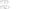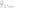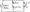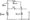# Network Analysis December 2010

Note: Answer any FIVE full questions.

1 a. Obtain the current source equivalent of a practical voltage source.

b.  In the network shown in Fig.Q1 (b), find the power delivered by the source, using the nodal analysis,

c.     Using mesh current analysis, find the current through the capacitor shown in Fig.Q1 (c).

2 a. State and prove the maximum power transfer theorem, for ac networks.

b.  Find the value of Rl shown in Fig.Q2(b) at which maximum power is transferred across ab. What is the maximum power transferred?Fig.Q2(b)        Fig.Q2(c)

c.   Find the current through the 6Q resistor shown in Fig.Q2(c), using the superposition theorem.

3 a. Obtain the Thevenin’s equivalent at terminals a-b shown in Fig.Q3(a).Fig. Q3 (a)

b.   State the reciprocity theorem.

c. Using the Millman’s theorem, find the current through the 10 Q resistor shown in Fig.Q3(c).

4 a. Define the following with respect to a graph:

i) Loop      ii) Cut set          iii) Tree              iv) Co-tree

b.   For the graph shown in Fig.Q4(b), draw any two trees and corresponding co-trees.Fig. Q4(b)

c. Obtain the dual of the network shown in Fig.Q4(d). draw the graph.

5 a. Prove that a parallel R-L and R-C circuit can resonate at all frequencies. Derive the condition.

b.  In a series RLC circuit, R = 2 Q, L = 2.0 mtr, C = 10 jliF. Find the resonant frequency, Q factor, bandwidth and half power frequencies.

c.   Determine C for the network shown in Fig.Q5(c) for the network to resonate.Fig-Q5(c)

6 a. In the circuit shown in Fig.Q6(a), find the initial current through the inductor, iiXt) and the time when the current in the inductor reduces to half its original value. The switch is openedFig.Q 6(a)        Fig.Q 6(b)

b.  In the network shown in Fig.Q 6(b), obtain the expression for the voltage across the capacitor, if the switch is closed at t ~ 0.

7 a. For the network shown in Fig. Q7(a), draw the frequency domain equivalent network and solve for Vo(P) using Laplace transfers.In a series RL circuit an exponential voltage V = 50 e1001 (V) is applied at t = 0. R = 10 Q and L = 0.2 H. Evaluate the current using the Laplace transforms.

8 a. Obtain the z-parameters of a two port network in terms of its h-parameters.

b.  Find the Y parameters of the network shown in Fig.Q8 (b).

c.   Obtain the ABCD parameters of two networks connected in cascade.